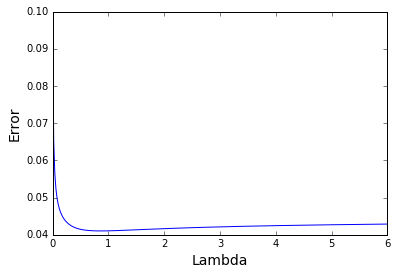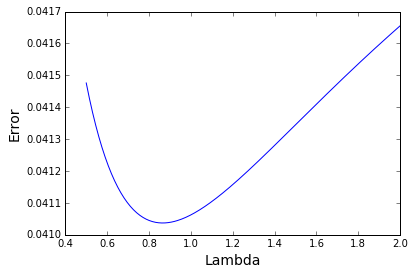# Grading the Individual Performances at the Ryder Cup

Ryder_Cup_2

Hooray! The U.S. won the Ryder Cup. A big relief for all the U.S. golf fans!

Quick aside: for those unfamiliar with the Ryder Cup, let me explain what it is. It is a unique golf event in that it is a team event. It has a long history; for the past 40 or so years it has been a competition between the U.S. and Europe. Each side fields 12 players - most of whom earn their spot and some of whom are chosen by a captain. The teams compete in 28 matches overall, 16 of which are two vs. two, and 12 of which are one vs. one.

Ok, now that that is out of the way, on to the interesting data stuff! I know what you're thinking, let's get to some code! Well, let's do it. I took some time and entered in the data from this year's Ryder Cup. Let's load it in.

In :
import pandas as pd
import numpy as np
import matplotlib.pyplot as plt
%matplotlib inline

In :
data = pd.read_csv('./../data/RyderCup/RyderCup.csv')

In :
data.iloc[0:2,np.array([4,7,20,22,24])]

Out:
Rory McIlroy Henrik Stenson Patrick Reed Jordan Spieth Result
0 -1 0 1 0 1 up
1 0 1 0 -1 3 and 2

The data has 28 rows - one for each match - and 25 columns - one for each player plus one results column. The data is coded in the following fashion: since Patrick Reed beat Rory McIlroy in a singles match with the final result 1 up, Patrick Reed's column get's a positive one, Rory McIlroy's columns gets a negative one, and '1 up' is in the Result column. The reasons behind these choices will become clear once I reveal the motivation (which you might've surmised already from the title).

My motivation is to determine who had the best individual performance during the Ryder Cup. The Ryder Cup is absolutley a team event. However, golf fans love to discuss how each player performed as an individual. Hence golf writers will write plenty of articles over the next 24 hours to 'grade' the individual players on their performance based on their own expert (subjective) opinions. My motivation is simply to use cold hard numbers to grade the individual performances of the players.

Before we can get into the math, I will need to state my assumption. Here it is: all of a player's performance can be adequetly represented by one number. So we cannot say that Spieth played especially well on Friday but poorly on the weekend, instead we just say he played this well over the entire three days of the Cup.

Moreover, these numbers add together to determine the results of the matches. For player A vs. player B, the result of the match is determined by player A's skill - player B's skill + some irreducible error. And for player A and player B vs. player C and player D, the result of the match is determined by player A's skill + player B's skill - (player C's skill + player D's skill) + some irreducible error. This assumption is probably not perfect but we don't have enough data to use anything else but a very simple model.

Now, hopefully you can see why I entered the data in this fashion. (We have it set up for a nice, simple, linear algebra problem.) First before we can go further, we need to do something with this 'Result' column. I will need to convert it to a number. I will do this by dividing the margin of victory (in holes) by the number of holes the match was contested over. For example, for those unfamiliar with golf, '1 up' means the margin of victory was 1 hole and the match went a full 18 holes. Thus the result would be 1/18. This represents a close match. At the opposite end of the spectrum would be the most lopsided result of this years' Cup - '5 and 4'. This means the margin of victory was 5 and the match ended after the 14th hole (the 4 means that there were 4 holes left). This will be encoded as 5/14. And finally, ties will be encoded as 0. Let's do this now.

In :
def convert_results(result):
if result=='1 up':
return 1/18.0
elif result=='halved':
return 0.0
else:
return float(result.split(' and '))/(18-float(result.split(' and ')))

In :
data.Result = [convert_results(result) for result in data.Result]
data.iloc[0:2,np.array([4,7,20,22,24])]

Out:
Rory McIlroy Henrik Stenson Patrick Reed Jordan Spieth Result
0 -1 0 1 0 0.055556
1 0 1 0 -1 0.187500

Now we can get to the fun part - figuring out the 'skill coefficients'. Using this additive model that assigns one number to each player's overall performance during the Cup, we now have a system of equations. We have 28 equations and 24 unknown variables. The form of the equation is:

$Ax = b$

and the system is over-determined, meaning we have more equations than unknowns. However, if we multiply both sides of the equation by $A^T$, we will get the square matrix $A^TA$ on the left side of the equation.

$A^TAx = A^Tb$

We can then solve the equation by computing the inverse - $(A^TA)^{-1}$ - and multiplying both sides by this matrix. But wait a minute, is that matrix even invertible? Let's compute its rank..

Making the matrix and vector:

In :
A = data.values[:,:-1].astype(float)
b = data.Result.values
print A.shape,b.shape

(28, 24) (28,)

In :
import numpy as np
np.matmul(A.T,A).shape, np.linalg.matrix_rank(np.matmul(A.T,A))

Out:
((24, 24), 23)

Uh-oh. Our matrix is not invertible! What ever will we do? Well here is one strategy: we compute the psuedo-inverse of the matrix instead of the inverse. This will give us the 'best' solution in terms of minimizing squared error of the resulting solution (reference).

$\hat{x} = (A^TA)^\ast * A^Tb$

Here it is in code:

In :
x_hat = np.dot(np.matmul(np.linalg.pinv(np.matmul(A.T,A)),A.T),b)


Now let's see how much error there is for each of the 28 equations. I'll print out the top five absolute errors.

In :
b_hat = np.dot(A,x_hat)
np.sort(np.abs(b_hat - b))[::-1][:5]

Out:
array([ 0.33981092,  0.20483193,  0.13497899,  0.07612017,  0.06746447])

Something to keep in mind here is that the above is the 'training' error rather than the testing error. The coefficients were found using the same data that we used to calculate the erorr.

Let's take a look at the coefficients that we just found. I'll print out the highest 5 and the lowest 5.

In :
np.sort(x_hat)[::-1][:5],np.sort(x_hat)[:5]

Out:
(array([ 0.67794105,  0.41127438,  0.31878228,  0.25259497,  0.23203036]),
array([-0.48459143, -0.43769157, -0.29833906, -0.28196394, -0.27055598]))

The estimated coefficients are very high. By this measure, if one were to replace the worst player with the best player, a team could go from tying the match, to winning every hole. This seems unreasonable.

So, let's think about how we arrived at this result. We chose the estimate that would minimize the sum of the squared error of the matches. Essentially, we performed Least Squares Regression with all of our variables with no form of regularization. This probably was not a very smart idea. If we were being bayesian, we would have a pretty strong prior on the fact that all the coefficients are pretty close to zero. Replacing one player with another at the highest level of golf is not that big of a deal. If we had some sort of pro-am, that would be a different story.

Anyway, how can we use regularization to get more reasonable results? We can penalize the squared sum of the coefficients and shrink them closer to zero. As we will see, this will actually improve the value of the coefficients in terms of predicting data that has not been seen.

There's also an added benefit of adding regularization - it makes our unsolvable systems of equations before - $A^TAx = A^Tb$ - solvable. This is because the new system of equations is this:

$(A^TA + \lambda I)x = A^Tb$

where $\lambda$ is a shrinkage coefficient for use to choose. Let's see what I mean by computing the rank of this matrix $(A^TA + \lambda I)$ with $\lambda = 1$ ..

In :
np.matmul(A.T,A).shape, np.linalg.matrix_rank(np.matmul(A.T,A) + np.eye(np.matmul(A.T,A).shape))

Out:
((24, 24), 24)

Now we've got a solvable system of equations! We are left with just one issue - how do we choose $\lambda$? This shrinkage parameter is normally chosen using cross validation. Luckily for us, we are using a linear model so instead of having to fit models over and over again, we can use a shortcut!

For linear models, the leave-one-out cross-validation, defined as

$CV = \frac{1}{N} \sum_{i=1}^{N} \hat{y}_{(-i)} - y_{i}$ where $\hat{y}_{(-i)}$ is the prediction of the ith observation using a model fit with all observations expect the ith and $y_{i}$ is the true value of the ith observation,

is equal to $\frac{1}{N} \sum_{i=1}^{N} [\frac{y_{i} - \hat{y_{i}}}{(1-h_{i})}]^2$ where $\hat{y_{i}}$ is the prediction of the ith observation using the full model and $h_i$ is the ith value on the diagonal of the 'hat' matrix which in our case is $A(A^TA + \lambda I)^{-1}A^T$.

This magical formula will allow us to do less work! All we have to do is compute that leave-one-out error for different values of lambda and then choose the lambda that gives us the smallest leave-one-out error! Let's get to it - I'll make a function that will take a value for lambda (I'll use 'lamb' since 'lambda' is reserved in Python) and spit out a value for the leave-one-out error.

In :
def loo_error(lamb):
a_t_a = np.matmul(A.T,A)
hat = np.matmul(np.matmul(A,np.linalg.inv(a_t_a + lamb*np.eye(24))),A.T)
x_hat = np.dot(np.matmul(np.linalg.inv(a_t_a + lamb*np.eye(24)),A.T),b)
b_hat = np.dot(A,x_hat)
error = 1/24. * np.sum(((b-b_hat) / (1-np.diag(hat)))**2)
return error


Yikes, that code got pretty hairy! Now let's try some different values for lambda! I'll try a broad range and then plot the results.

In :
plt.plot(np.linspace(.0001,6,300),[loo_error(lamb) for lamb in np.linspace(.0001,6,300)]);
plt.xlabel('Lambda',fontsize = 14);
plt.ylabel('Error',fontsize = 14);Let's zoom in to the area of interest.

In :
plt.plot(np.linspace(.5,2,300),[loo_error(lamb) for lamb in np.linspace(.5,2,300)]);
plt.xlabel('Lambda',fontsize = 14);
plt.ylabel('Error',fontsize = 14);Awesome, we see our min is around .85. Let's get the lambda that corresponds to the min value and then compute those coefficients!

In :
lamb = np.linspace(.5,2,300)[np.argmin([loo_error(lamb) for lamb in np.linspace(.5,2,300)])]
lamb

Out:
0.86622073578595327
In :
x_hat = np.dot(np.matmul(np.linalg.inv(np.matmul(A.T,A) + lamb*np.eye(24)),A.T),b)

In :
result = pd.DataFrame({'player':data.columns[:-1],'coefficient':x_hat})
result = result[['player','coefficient']].sort_values('coefficient',ascending=False)
result.index = range(1,25)
result

Out:
player coefficient
1 Zach Johnson 0.143236
2 Rory McIlroy 0.116069
3 Patrick Reed 0.096376
4 Brooks Koepka 0.089798
5 Rafa Cabrera Bello 0.079656
6 Thomas Pieters 0.072940
7 Brandt Snedeker 0.071616
8 Henrik Stenson 0.051752
9 Matt Kuchar 0.043422
10 Rickie Fowler 0.029276
11 Dustin Johnson 0.028538
12 Chris Wood 0.006250
13 Justin Rose -0.001379
14 Martin Kaymer -0.018094
15 Phil Mickelson -0.020971
16 Jimmy Walker -0.040542
17 Sergio Garcia -0.058348
18 Jordan Spieth -0.068327
19 Matt Fitzpatrick -0.070221
20 Ryan Moore -0.080695
21 J.B. Holmes -0.090542
22 Andy Sullivan -0.093564
23 Danny Willet -0.134506
24 Lee Westwood -0.151739

## Conclusion¶

This was fun but I do not pretend that this result is the definitive answer to who had the best individual Ryder Cup. There is very little data so there is not much to work with. Also, one thing to keep in mind is that these results are normalized for how many matches you played in. Many sports people would say yes Zach Johnson did great with a 2 wins and 0 loses, but Patrick Reed played 5 matches going 3-1-1 not to mention the emotional leadership that he showed. So this is definetly a caviot to the results. Nevertheless, I hope you enjoyed my analysis and thanks for reading!

Written on October 3, 2016